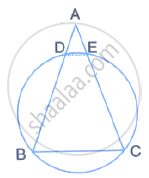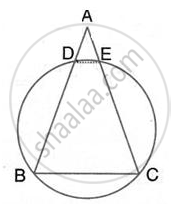Share

In the Following Figure Ab = Ac. Prove that Decb is an Isosceles Trapezium. - ICSE Class 10 - Mathematics

Question

In the following figure AB = AC. Prove that DECB is an isosceles trapezium.SolutionHere, AB = AC
⇒ ∠B =∠C
∴ DECB is a cyclic quadrilateral
(Ina triangle, angles opposite to equal sides are equal)
Also, ∠B + ∠DEC = 180°   ………. (1)
(pair of opposite angles in a cyclic quadrilateral are supplementary)
⇒∠C + ∠DEC = 180°  [from (1)]

But this is the sum of interior angles

On one side of a transversal.

∴ DE || BC But  ∠ADE = ∠AED = ∠C [Corresponding angles]

Thus, ∠ADE = ∠AED